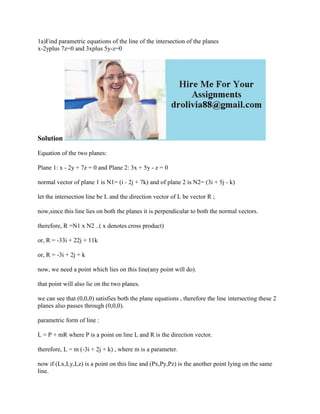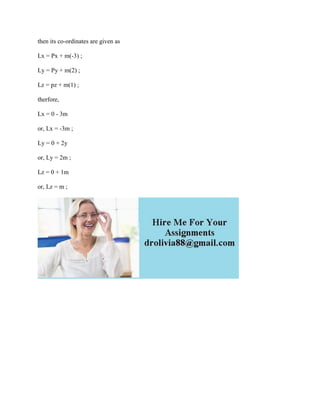Successfully reported this slideshow.

# 1a)Find parametric equations of the line of the intersection of the pl (2).docx×

# 1a)Find parametric equations of the line of the intersection of the pl (2).docx

1a)Find parametric equations of the line of the intersection of the planes
x-2yplus 7z=0 and 3xplus 5y-z=0
Solution
Equation of the two planes:
Plane 1: x - 2y + 7z = 0 and Plane 2: 3x + 5y - z = 0
normal vector of plane 1 is N1= (i - 2j + 7k) and of plane 2 is N2= (3i + 5j - k)
let the intersection line be L and the direction vector of L be vector R ;
now,since this line lies on both the planes it is perpendicular to both the normal vectors.
therefore, R =N1 x N2 ..( x denotes cross product)
or, R = -33i + 22j + 11k
or, R = -3i + 2j + k
now, we need a point which lies on this line(any point will do).
that point will also lie on the two planes.
we can see that (0,0,0) satisfies both the plane equations , therefore the line intersecting these 2 planes also passes through (0,0,0).
parametric form of line :
L = P + mR where P is a point on line L and R is the direction vector.
therefore, L = m (-3i + 2j + k) , where m is a parameter.
now if (Lx,Ly,Lz) is a point on this line and (Px,Py,Pz) is the another point lying on the same line.
then its co-ordinates are given as
Lx = Px + m(-3) ;
Ly = Py + m(2) ;
Lz = pz + m(1) ;
therfore,
Lx = 0 - 3m
or, Lx = -3m ;
Ly = 0 + 2y
or, Ly = 2m ;
Lz = 0 + 1m
or, Lz = m ;
.

1a)Find parametric equations of the line of the intersection of the planes
x-2yplus 7z=0 and 3xplus 5y-z=0
Solution
Equation of the two planes:
Plane 1: x - 2y + 7z = 0 and Plane 2: 3x + 5y - z = 0
normal vector of plane 1 is N1= (i - 2j + 7k) and of plane 2 is N2= (3i + 5j - k)
let the intersection line be L and the direction vector of L be vector R ;
now,since this line lies on both the planes it is perpendicular to both the normal vectors.
therefore, R =N1 x N2 ..( x denotes cross product)
or, R = -33i + 22j + 11k
or, R = -3i + 2j + k
now, we need a point which lies on this line(any point will do).
that point will also lie on the two planes.
we can see that (0,0,0) satisfies both the plane equations , therefore the line intersecting these 2 planes also passes through (0,0,0).
parametric form of line :
L = P + mR where P is a point on line L and R is the direction vector.
therefore, L = m (-3i + 2j + k) , where m is a parameter.
now if (Lx,Ly,Lz) is a point on this line and (Px,Py,Pz) is the another point lying on the same line.
then its co-ordinates are given as
Lx = Px + m(-3) ;
Ly = Py + m(2) ;
Lz = pz + m(1) ;
therfore,
Lx = 0 - 3m
or, Lx = -3m ;
Ly = 0 + 2y
or, Ly = 2m ;
Lz = 0 + 1m
or, Lz = m ;
.